# R S Aggarwal Solutions for Class 10 Maths Chapter 7 Triangles Exercise 7C

R S Aggarwal Solutions for class 10 Maths chapter 7 exercise 7C is available here. This set of important questions helps students to master triangles problems. This exercise is based on the ratio of the areas of two similar triangles. All questions are solved by subject experts at BYJU’S. Students can freely download R S Aggarwal Class 10 chapter 7 and practice more questions.

## Download PDF of R S Aggarwal Solutions for Class 10 Maths Chapter 7 Triangles Exercise 7C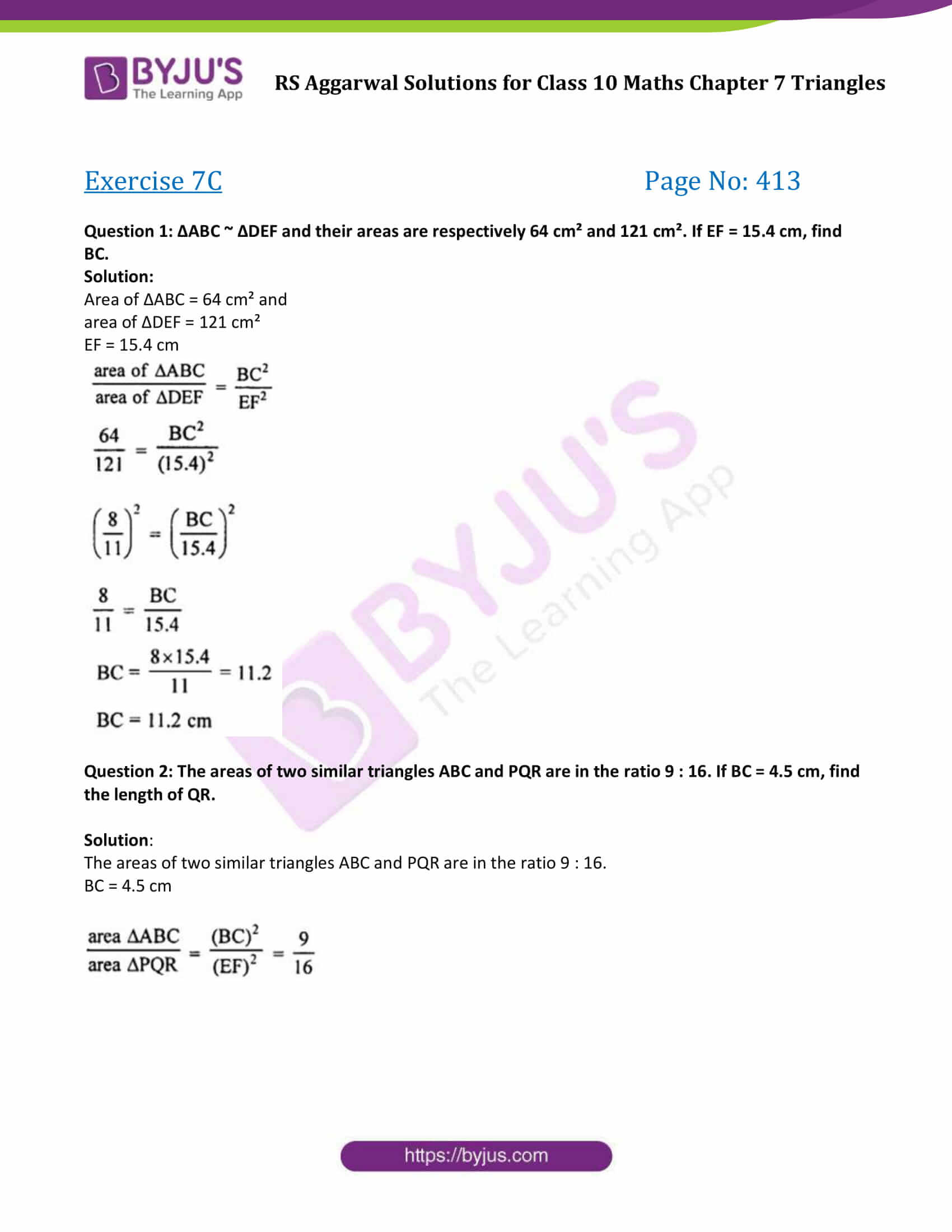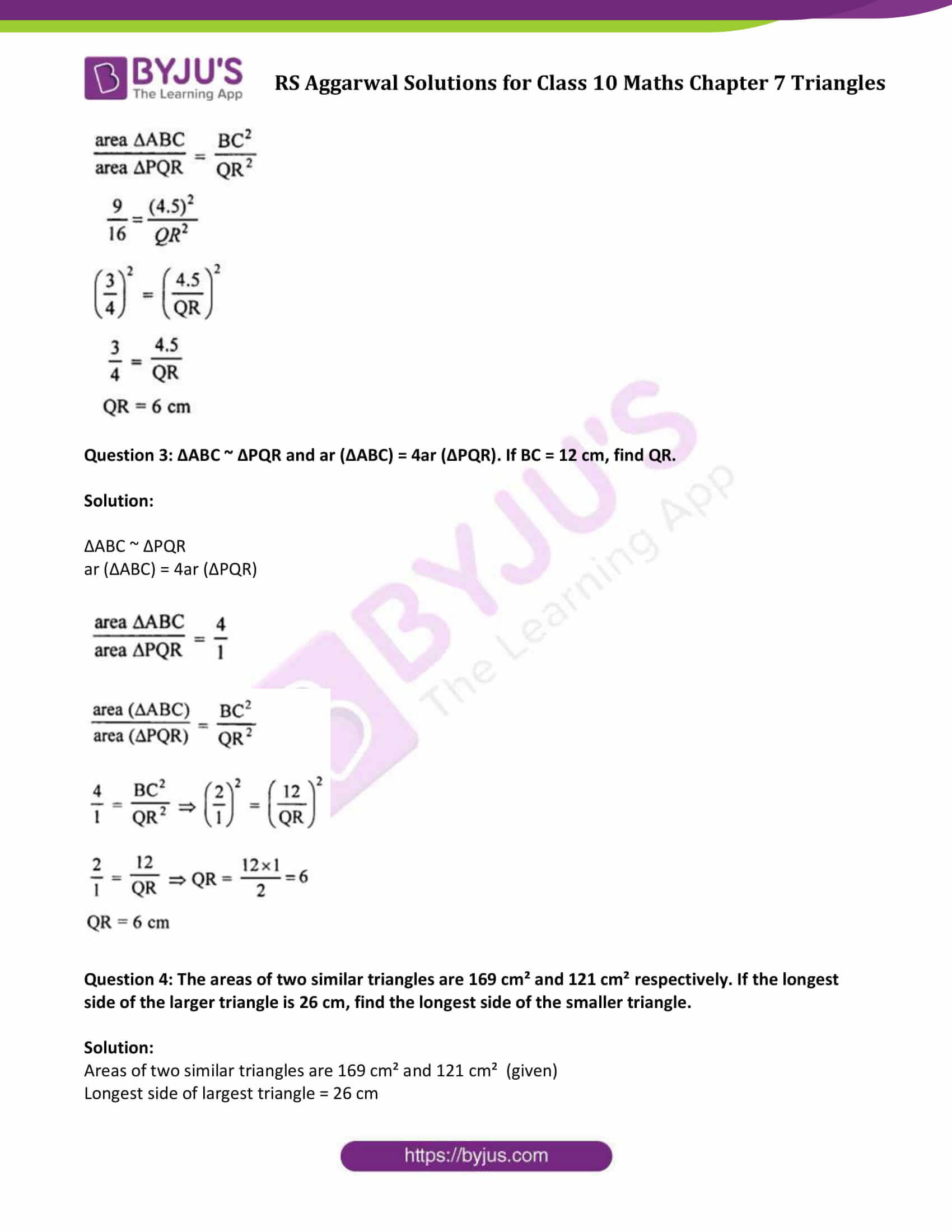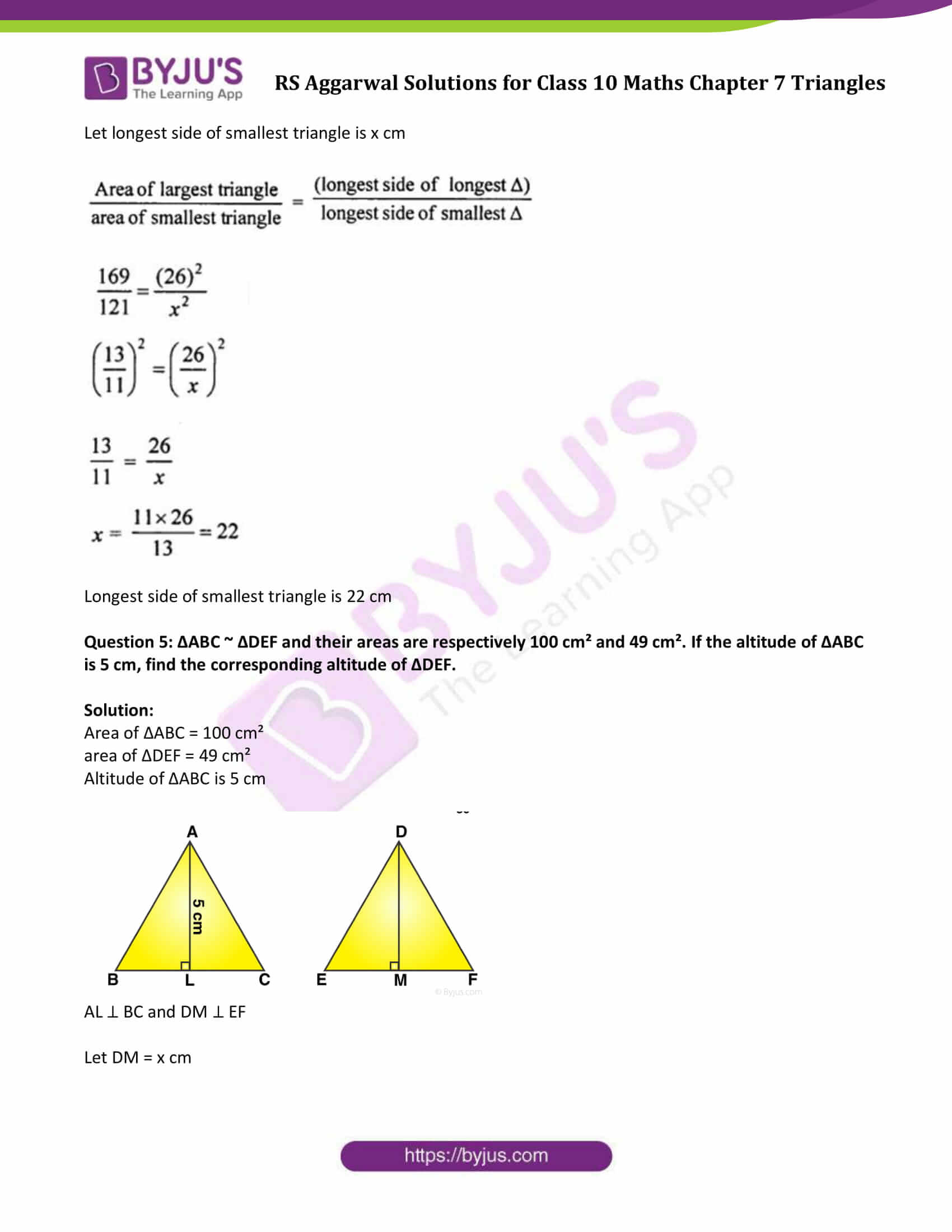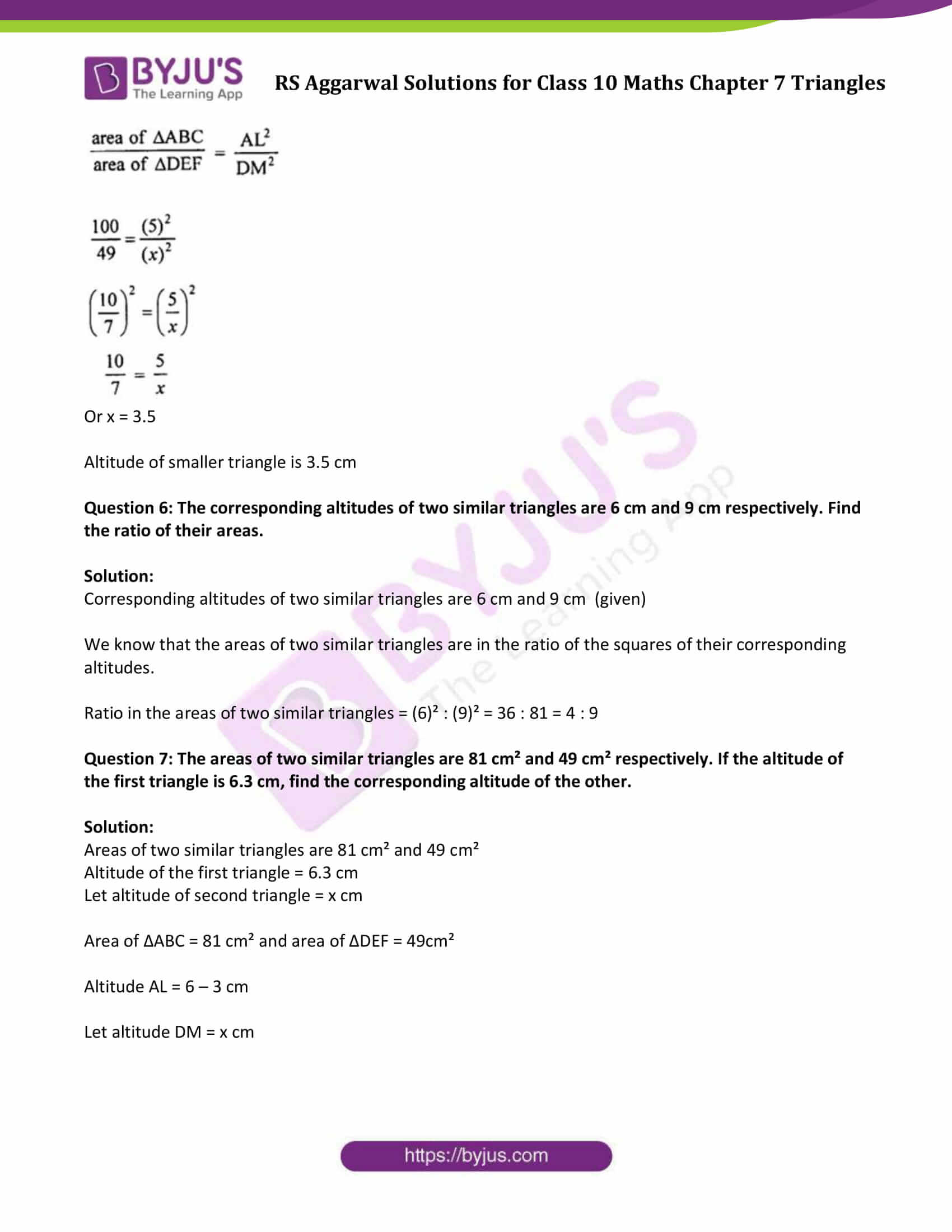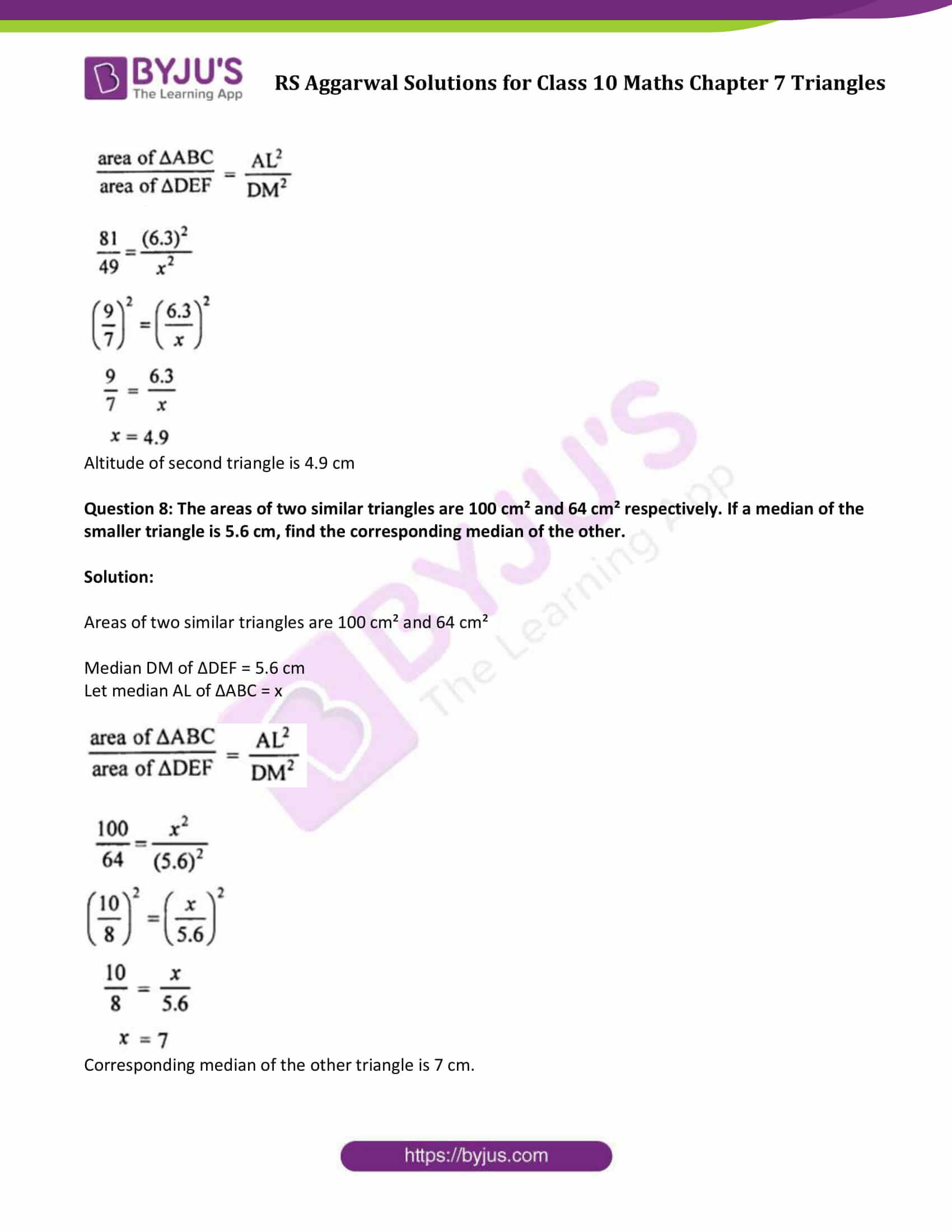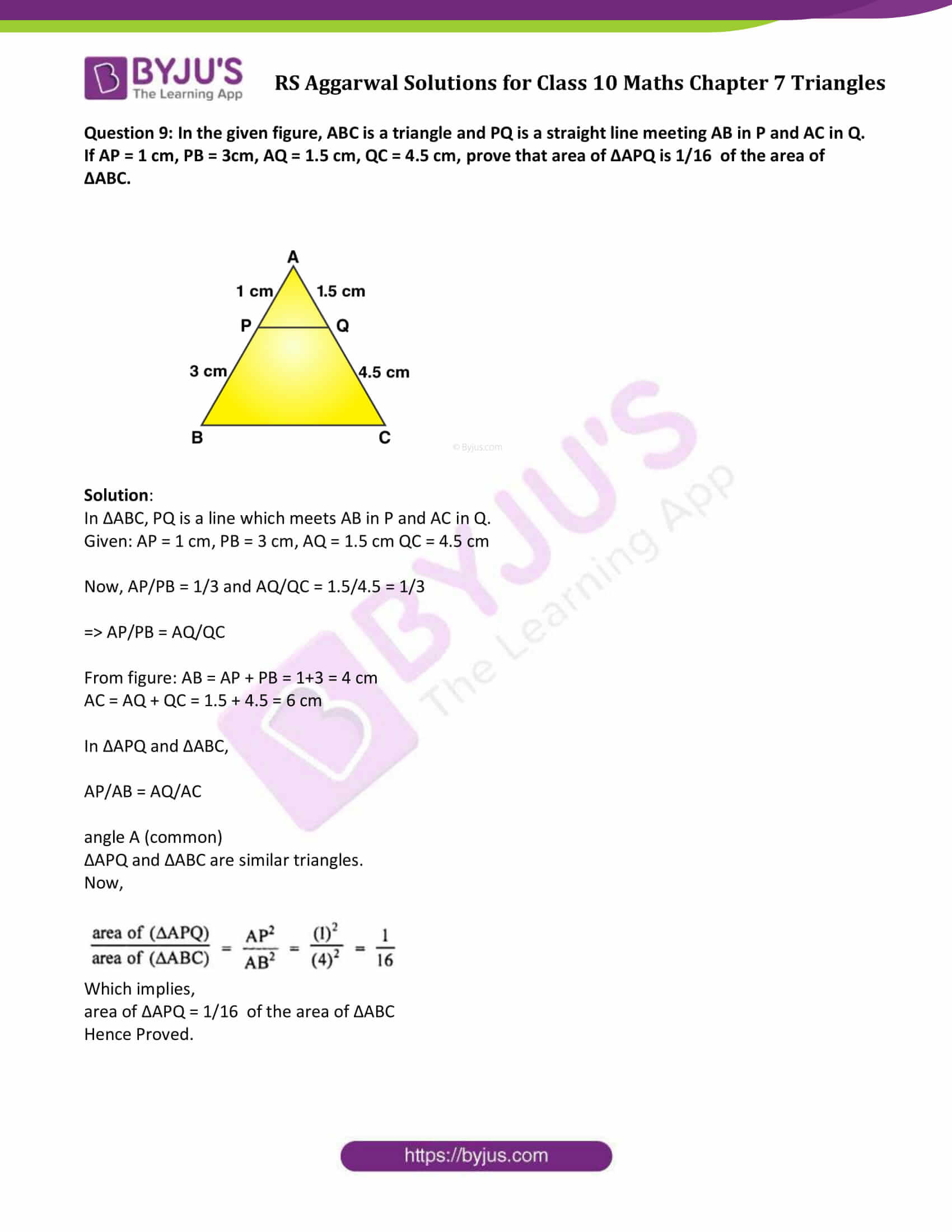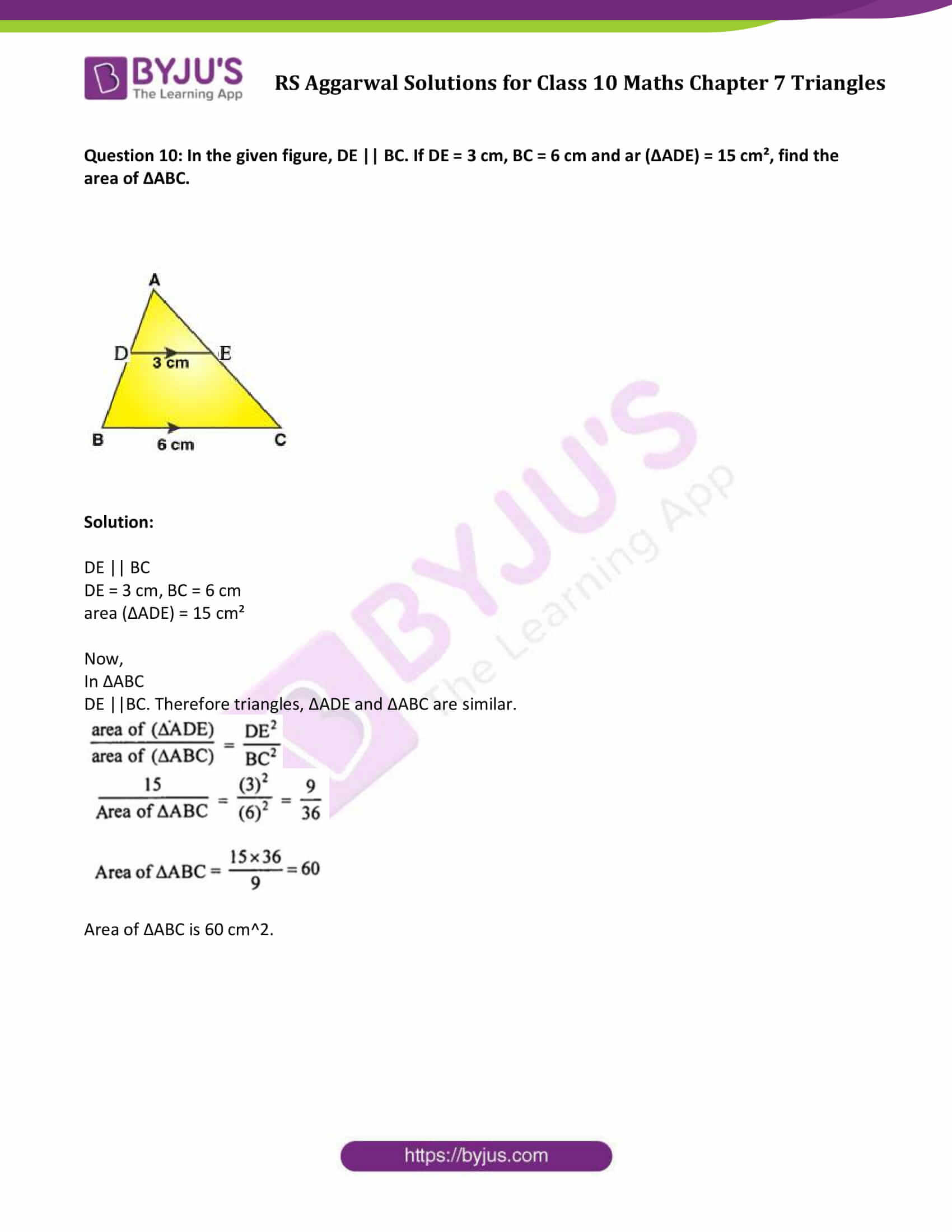### Access other exercise solutions of Class 10 Maths Chapter 7 Triangles

Exercise 7 A Solutions

Exercise 7 B Solutions

Exercise 7 D Solutions

Exercise 7 E Solutions

Question 1: ∆ABC ~ ∆DEF and their areas are respectively 64 cm² and 121 cm². If EF = 15.4 cm, find BC.

Solution:

Area of ∆ABC = 64 cm² and

area of ∆DEF = 121 cm²

EF = 15.4 cm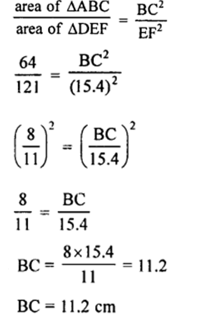Question 2: The areas of two similar triangles ABC and PQR are in the ratio 9 : 16. If BC = 4.5 cm, find the length of QR.

Solution:

The areas of two similar triangles ABC and PQR are in the ratio 9 : 16.

BC = 4.5 cm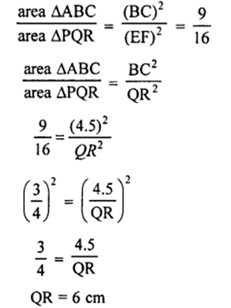Question 3: ∆ABC ~ ∆PQR and ar (∆ABC) = 4ar (∆PQR). If BC = 12 cm, find QR.

Solution:

∆ABC ~ ∆PQR

ar (∆ABC) = 4ar (∆PQR)Question 4: The areas of two similar triangles are 169 cm² and 121 cm² respectively. If the longest side of the larger triangle is 26 cm, find the longest side of the smaller triangle.

Solution:

Areas of two similar triangles are 169 cm² and 121 cm² (given)

Longest side of largest triangle = 26 cm

Let longest side of smallest triangle is x cm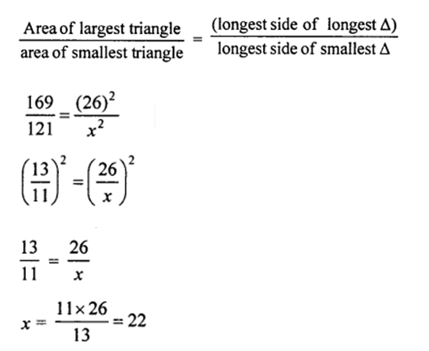Longest side of smallest triangle is 22 cm

Question 5: ∆ABC ~ ∆DEF and their areas are respectively 100 cm² and 49 cm². If the altitude of ∆ABC is 5 cm, find the corresponding altitude of ∆DEF.

Solution:

Area of ∆ABC = 100 cm²

area of ∆DEF = 49 cm²

Altitude of ∆ABC is 5 cm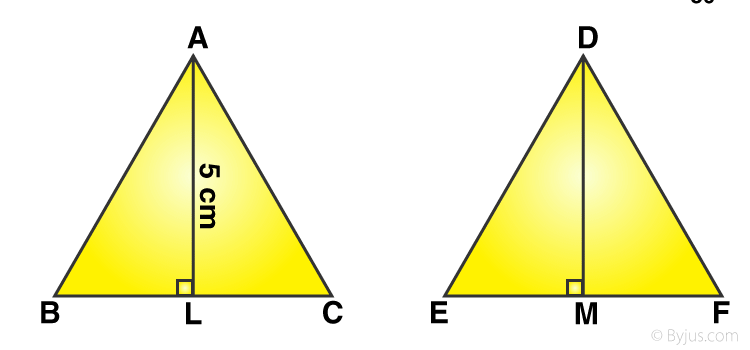AL ⊥ BC and DM ⊥ EF

Let DM = x cm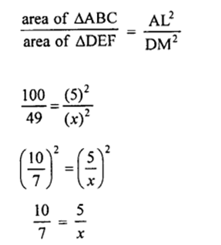Or x = 3.5

Altitude of smaller triangle is 3.5 cm

Question 6: The corresponding altitudes of two similar triangles are 6 cm and 9 cm respectively. Find the ratio of their areas.

Solution:

Corresponding altitudes of two similar triangles are 6 cm and 9 cm (given)

We know that the areas of two similar triangles are in the ratio of the squares of their corresponding altitudes.

Ratio in the areas of two similar triangles = (6)² : (9)² = 36 : 81 = 4 : 9

Question 7: The areas of two similar triangles are 81 cm² and 49 cm² respectively. If the altitude of the first triangle is 6.3 cm, find the corresponding altitude of the other.

Solution:

Areas of two similar triangles are 81 cm² and 49 cm²

Altitude of the first triangle = 6.3 cm

Let altitude of second triangle = x cm

Area of ∆ABC = 81 cm² and area of ∆DEF = 49cm²

Altitude AL = 6 – 3 cm

Let altitude DM = x cm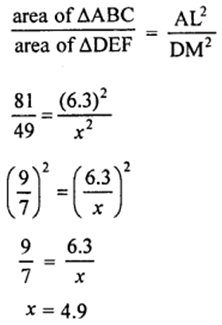Altitude of second triangle is 4.9 cm

Question 8: The areas of two similar triangles are 100 cm² and 64 cm² respectively. If a median of the smaller triangle is 5.6 cm, find the corresponding median of the other.

Solution:

Areas of two similar triangles are 100 cm² and 64 cm²

Median DM of ∆DEF = 5.6 cm

Let median AL of ∆ABC = x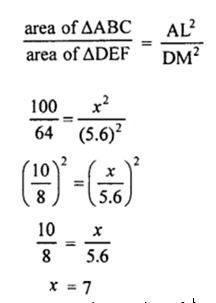Corresponding median of the other triangle is 7 cm.

Question 9: In the given figure, ABC is a triangle and PQ is a straight line meeting AB in P and AC in Q. If AP = 1 cm, PB = 3cm, AQ = 1.5 cm, QC = 4.5 cm, prove that area of ∆APQ is 1/16 of the area of ∆ABC.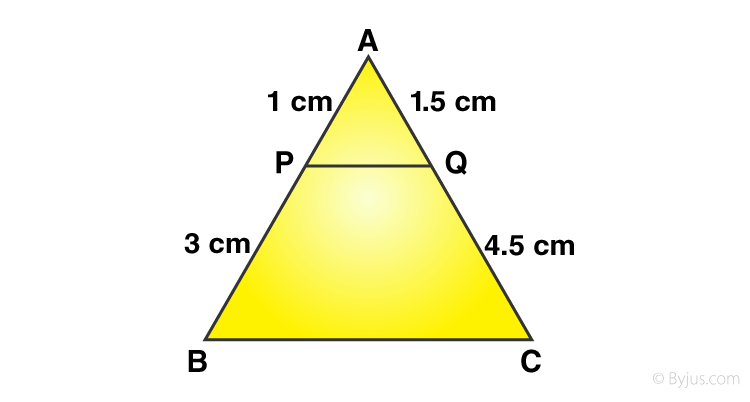Solution:

In ∆ABC, PQ is a line which meets AB in P and AC in Q.

Given: AP = 1 cm, PB = 3 cm, AQ = 1.5 cm QC = 4.5 cm

Now, AP/PB = 1/3 and AQ/QC = 1.5/4.5 = 1/3

=> AP/PB = AQ/QC

From figure: AB = AP + PB = 1+3 = 4 cm

AC = AQ + QC = 1.5 + 4.5 = 6 cm

In ∆APQ and ∆ABC,

AP/AB = AQ/AC

angle A (common)

∆APQ and ∆ABC are similar triangles.

Now,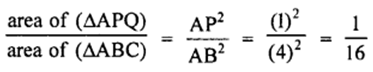Which implies,

area of ∆APQ = 1/16 of the area of ∆ABC

Hence Proved.

Question 10: In the given figure, DE || BC. If DE = 3 cm, BC = 6 cm and ar (∆ADE) = 15 cm², find the area of ∆ABC.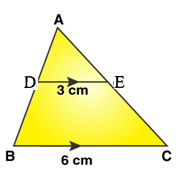Solution:

DE || BC

DE = 3 cm, BC = 6 cm

Now,

In ∆ABC

DE ||BC. Therefore triangles, ∆ADE and ∆ABC are similar.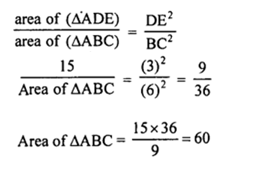Area of ∆ABC is 60 cm2.

## R S Aggarwal Solutions for Class 10 Maths Chapter 7 Triangles Exercise 7C

Class 10 Maths Chapter 7 Triangles Exercise 7C is based on the ratio of the areas of two similar triangles. The ratio of the areas of two similar triangles is equal to the ratio of the square of their corresponding sides.